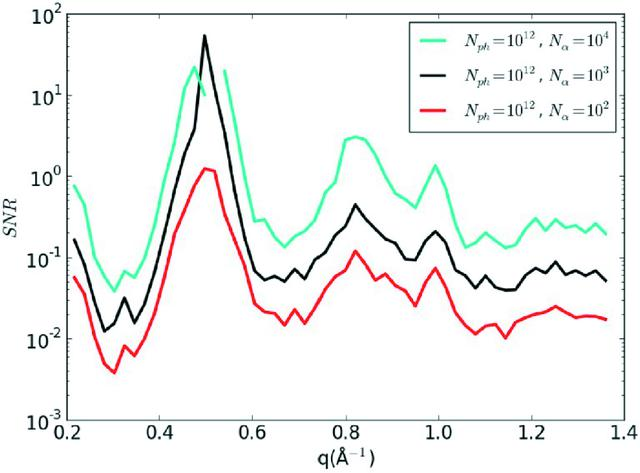disable zoom     view article Figure 5 The numerically calculated SNR as function of the number of patterns. The signal is the standard deviation of the noise-free calculation and the noise level is calculated from the difference between the variance of each noisy simulation and that of the noise-free simulation. The increase of the SNR is proportional to Nα1/2, as predicted by the theory in Appendix B.

IUCrJ
ISSN: 2052-2525